# Polynomials-Sample Questions

Class 10th Maths
Sample Paper for Polynomials
1.  For what value of k, (–4) is a zero of the polynomial x2 – x – (2k + 2)?
(CBSE 2009)
2.  For what value of p, (–4) is a zero of the polynomial x2 – 2x – (7p + 3)?
(CBSE 2009)
3.  If 1 is a zero of the polynomial p(x) = ax2 – 3(a – 1) x – 1, then find the value of a.
(Al CBSE 2009)
4.  If (x + a) is a factor of 2x2 + 2ax + 5x + 10 find a.
(Al CBSE 2008 F)
5.  Write the zeroes of the polynomial x2 + 2x + 1.
(CBSE 2008)
6.  Write the zeroes of the polynomial x2 – x – 6.
(CBSE 2008)
7.  Write a quadratic polynomial, the sum and product of whose zeroes are 3 and –2 respectively.
(CBSE 2008)
8.  Write the number of zeroes of the polynomial y = f(x) whose graph is given in the figure.
(Al CBSE 2008)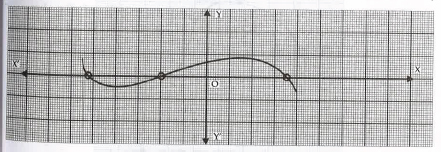9.  The graph of y = f(x) is given in figure. How many zeroes are there of f(x)?
(Al CBSE 2008 C)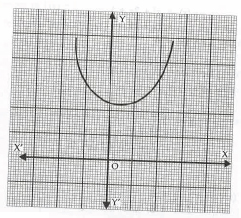10.  The graph of y = f(x) is given in the figure. What is the number of zeroes of f(x)?
(CBSE 2008 C)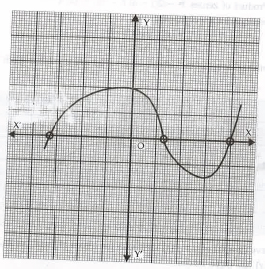11.  What is the number of zeroes of the polynomial y = p(x)?
(AI CBSE 2010)12.  Find the zeroes of the quadratic polynomial 6x2 – 3 – 7x and verify the relationship between the zeroes and the coefficient of the polynomial.
(CBSE 2008)
13.  Find the zeroes of the quadratic polynomial 5x2 – 4 – 8x and verify the relationship between the zeroes and the coefficient of the polynomial.
(AI CBSE 2008)
14.  Find the quadratic polynomial, the sum of whose zeroes is 8 and their product is 12. Hence, find the zeroes of the polynomial.
(CBSE 2008)
15.  If one zero of the polynomial (a2 – 9) x2 + 13x + 6a is reciprocal of the other, find the value of ‘a’.
(AI CBSE 2008)
16.  If the product of zeroes of the polynomial ax2 – 6x – 6 is 4, find the value of ‘a’.
(AI CBSE 2008)
17.  Find all the zeros of the polynomial x4 + x3 – 34x2 – 4x + 120, if two of its zeroes are 2 and – 2.
(AI CBSE 2008)
18.  Find all the zeroes of the polynomial 2x4 + 7x – 19x2 – 14x + 30, if two of its zeroes are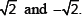(AI CBSE 2008)
19.  Find the quadratic polynomial whose zeroes are 1 and –3. Verify the relation between the coefficients and the zeroes of the polynomial.
(CBSE 2008 C)
20.  Find the zeroes of the quadratic polynomial 4x2 – 4x – 3 and verify the relation between the zeroes and its coefficients.
(CBSE 2008 C)
21.  Obtain all other zeroes of the polynomial 2x3 – 4x – x2 + 2, if two of its zeroes are(CBSE 2008 C)
22.  Find all the zeroes of x4 – 3x3 + 6x – 4, if two of its zeroes are(AI CBSE 2008 C)
23.  Find a quadratic polynomial whose zeroes are –4 and 3 and verify the relationship between the zeroes and the coefficients.
(AI CBSE 2008 C)
24.  Using division algorithm, find the quotient and remainder on dividing f(x) by g(x), where f(x) = 6x3 + 13x2 + x – 2 and g(x) = 2x + 1
(AI CBSE 2008 C)
25.  If the polynomial 6x4 + 8x3 + 17x2 + 21x + 7 is divided by another polynomial 3x2 + 4x + 1 then the remainder comes out to be ax + b, find ‘a’ and ‘b’
(CBSE 2009)
26.  If the polynomial x4 + 2x3 + 8x2 + 12x + 18 is divided by another polynomial x2 + 5, the remainder comes out to be px + q. Find the value of p and q.
(CBSE 2009)
27.  Find all the zeroes of the polynomial x3 + 3x2 – 2x – 6, if two of its zeroes are –(AI CBSE 2009)
28.  Find all the zeroes of the polynomial 2x3 + x2 – 6x – 3, if two of its zeroes are –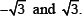(AI CBSE 2009)
29.  If α and β are zeroes of the quadratic polynomial x2 – 6x + a; find the value of ‘a’ if 3α + 2β = 20.
(CBSE 2010, 2011)